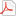## About Special Education at a Glance 2013-2014

### Guidelines for Reporting Data 2013-2014

The federal government has provided guidance regarding the Family Educational Rights and Privacy Act (FERPA) that has resulted in restrictions in the amount of student data that may be publically reported. FERPA (20 U.S.C. § 1232g; 34 CFR Part 99) prohibits the release of individually identifiable information to the public. The Maryland State Department of Education (MSDE) adopted the guidelines for the reporting of aggregate student data and Montgomery County Public Schools (MCPS) is implementing these guidelines as follows:

#### Student Enrollment Data

• Any percentage rates greater than or equal to 95.0% or less than or equal to 5.0% will be noted as ≥95.0% or ≤5.0%, respectively.
• If the percentage rate is greater than or equal to 95.0% or less than or equal to 5.0%, the corresponding number of students (N) will not be published.
• When the total N is less than 10, the corresponding N's and percentage rate will not be published.
• When the total N is between 10 and 20, only the percentage rate will be published, provided the percentage rate is not greater than or equal to 95.0% or less than or equal to 5.0%.
• When the total N is greater than 20, the corresponding N's and percentage rate will be published, provided the percentage rate is not greater than or equal to 95.0% or less than or equal to 5.0%.

#### Out-of-School Suspension Data

• Any percentage rates greater than or equal to 95.0% or less than or equal to 3.0% will be noted as ≥95.0% or ≤3.0%, respectively.
• If the percentage rate is greater than or equal to 95.0% or less than or equal to 3.0%, the corresponding number of students enrolled, number of suspension incidents, and number of students suspended will not be published.
• When the number of students enrolled is less than 10 or the number of students suspended is less than 5, no data will be published.
• When the number of students enrolled is between 10 and 20, only the percentage rate will be published, provided the percentage rate is not greater than or equal to 95.0% or less than or equal to 3.0%.
• When the number of students enrolled is greater than 20, the corresponding number of suspension incidents, number of students suspended, and percentage rate will be published, provided the percentage rate is not greater than or equal to 95.0% or less than or equal to 3.0%.

Download Guidelines for Reporting Data